# NCERT Solutions for Class 9 Maths Chapter 4 Exercise 4.4 – Linear Equations in two Variables

Download NCERT Solutions for Class 9 Maths Chapter 4 Exercise 4.4 – Linear Equations in two Variables. This Exercise contains 2 questions, for which detailed answers have been provided in this note. In case you are looking at studying the remaining Exercise for Class 9 for Maths NCERT solutions for Chapter 5 or other Chapters, you can click the link at the end of this Note.

### NCERT Solutions for Class 9 Maths Chapter 4 Exercise 4.4 – Linear Equations in two Variables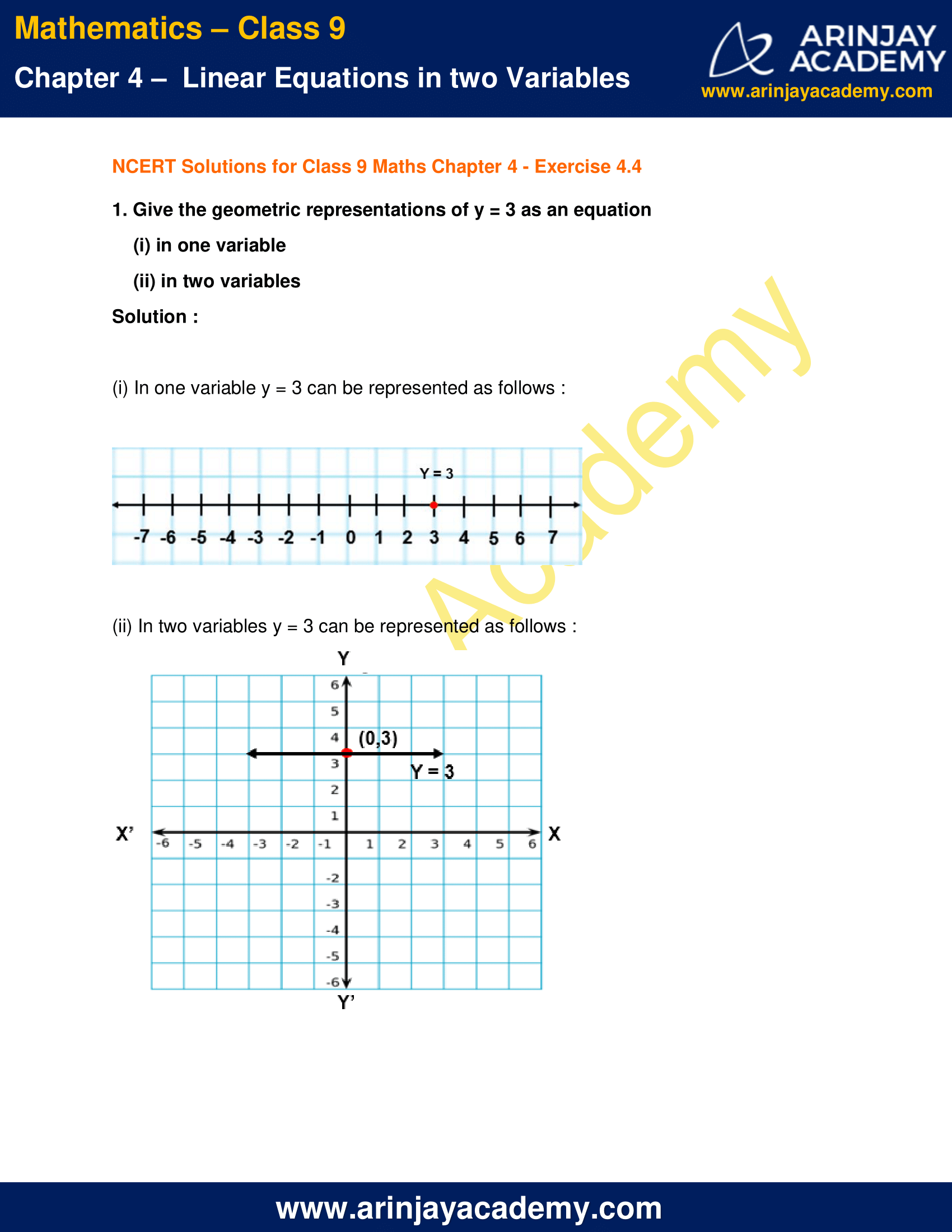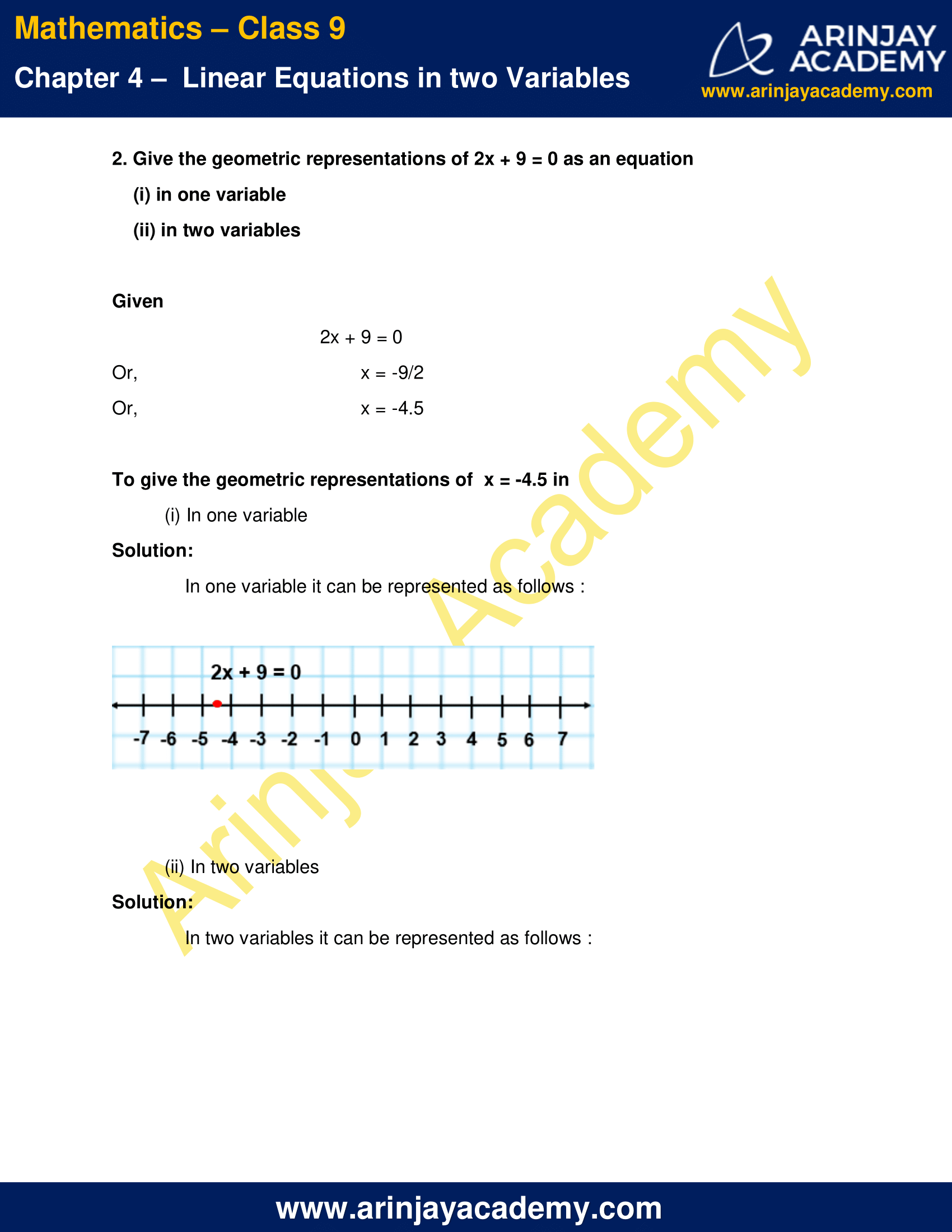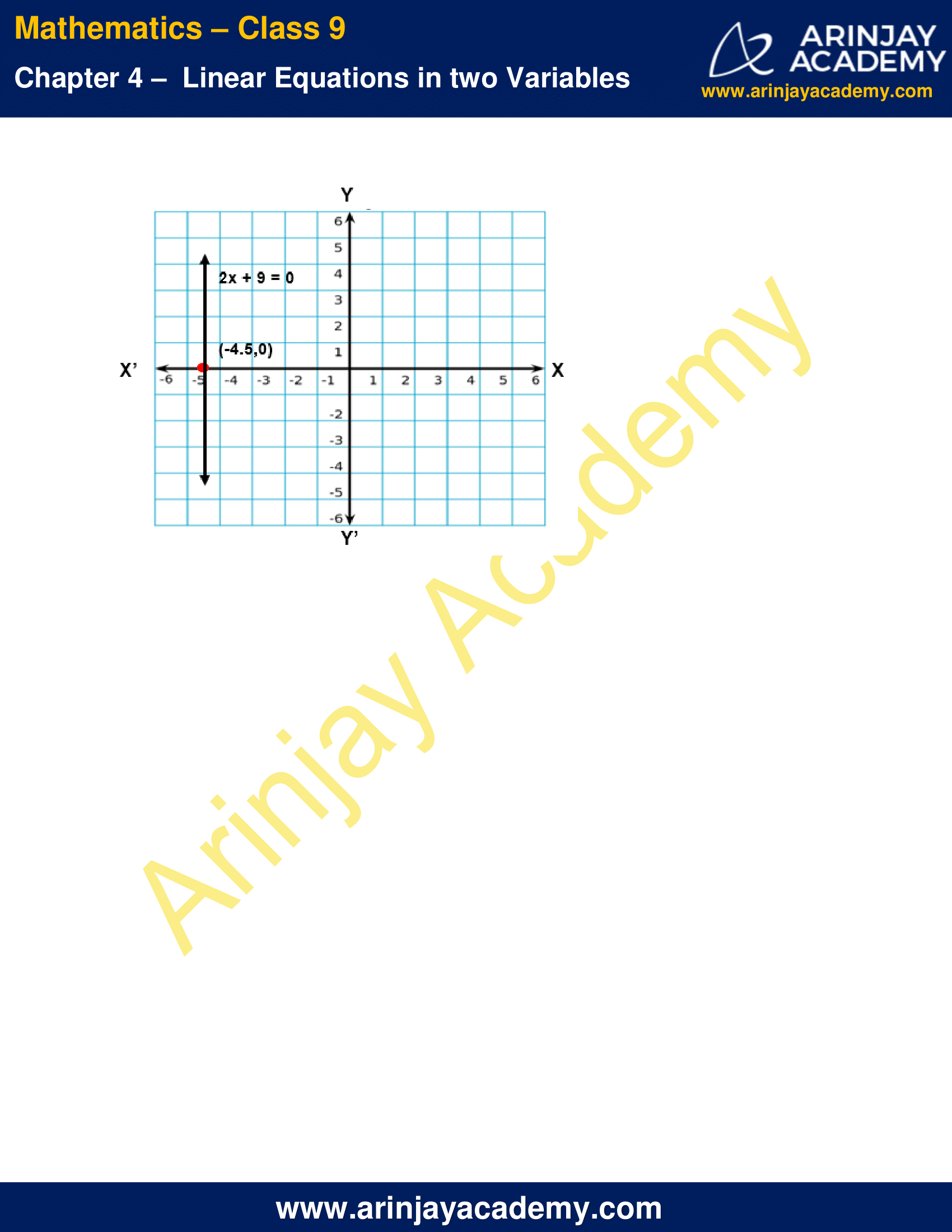NCERT Solutions for Class 9 Maths Chapter 4 Exercise 4.4 – Linear Equations in two Variables

1. Give the geometric representations of y = 3 as an equation

(i) in one variable
(ii) in two variables

Solution :

(i) In one variable y = 3 can be represented as follows :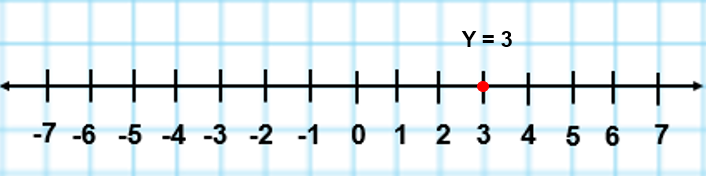(ii) In two variables y = 3 can be represented as follows :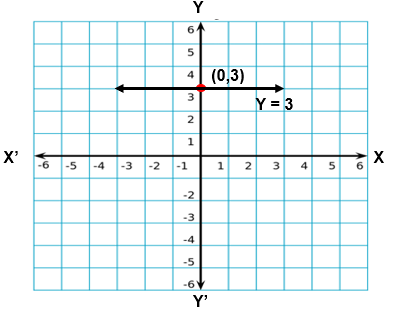2. Give the geometric representations of 2x + 9 = 0 as an equation

(i) in one variable
(ii) in two variables

Given

2x + 9 = 0
x = -9/2
x = -4.5

To give the geometric representations of  x = -4.5 in

(i) In one variable

Solution:

In one variable it can be represented as follows :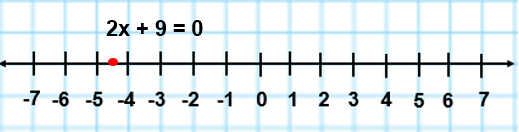(ii) In two variables

Solution:

In two variables it can be represented as follows :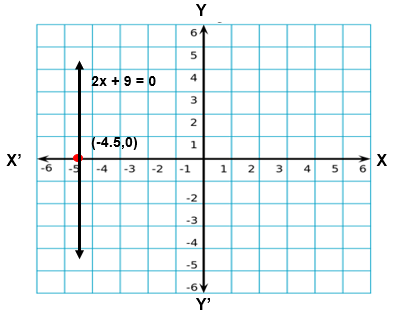NCERT Solutions for Class 9 Maths Chapter 4 Exercise 4.4 – Linear Equations in two Variables, has been designed by the NCERT to test the knowledge of the student on the topic – Equations of Lines Parallel to the x-axis and y-axis

The next Exercise for NCERT Solutions for Class 9 Maths Chapter 5 Exercise 5.1 – Introduction to Euclid’s Geometry can be accessed by clicking here.

Download NCERT Solutions for Class 9 Maths Chapter 4 Exercise 4.4 – Linear Equations in two Variables